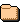# index.html

### Browse the distribution tree..

### Sub Directories..debugeh3sycrlaowinvmjxkPackagesescrlaowinvmxkgdpqtfbugdpqtfb4uzPackagesehsycrlaowinvmjxkgdpqtfbuz